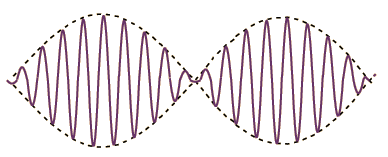# Beat Frequency Formula

The beat is a word used for sound waves. The beat frequency is the difference in frequency of two waves. It is because of constructive and destructive interference. In sound, we hear said beat frequency as the rate at which the loudness of the sound varies whereas we hear the ordinary frequency of the waves as the pitch of the sound. Here we discuss the concept of beat frequency and beat frequency formula in detail.

## What is Beat Frequency?

When two waves of nearly equal frequencies traveling in a medium along the same direction meet at a point, beats are produced. When two sound waves of dissimilar frequency approach your ear, the alternating productive and damaging interference causes the sound to be alternatively loud and soft this is a phenomenon, which is termed as beating.The beat frequency is equal to the complete value of the alteration in the frequency of the two waves. The count of beats per second is equivalent to the difference in frequencies of two waves is called beat frequency.

### Beat Frequency Formula:

fb = $$\left | f_2 – f_1 \right |$$

Where,

 fb Beat frequency f1 Frequency of 1st  wave f2 Frequency of 2nd wave

### Derivation of Beat Frequency Formula:

Consider sound from two different sources whose medium is air is meeting at a point p. Let us assume that one source has a shorter time period as TS and high frequency as f2 whereas other source time periods and frequency are TL and f1. With the help of these frequencies, we represent beat frequency by showing the relationship between these two frequencies.

As in our conceptual discussion, let us start at an instant when a crest from each source is at point p. When, after an amount of time TS  passes, the next crest from the shorter-period source arrives, the corresponding crest from the longer-period source won’t arrive for an amount of time $$Delta T =T_L − T_S$$. In fact, with the arrival of each successive short-period crest, the corresponding long-period crest is another $$\Delta$$ T behind. Eventually, after some number n of short periods, the long-period crest will arrive a full long period TL after the corresponding short period crest arrives.

$$n \times \Delta T = T_L$$- (1)

This means that as the short-period crest arrives, the long-period crest that precedes the corresponding long-period crest is arriving. This results in constructive interference (loud sound). The time it takes, starting when the interference is maximally constructive, for the interference to become maximally constructive is the beat period

$$T_{BEAT}=n \times T_S$$  – (2)

From 1 & 2, We get

$$T_{BEAT}= \frac{T_L}{\Delta T}$$ TS

Here $$\Delta T = T_L – T_S$$

So, $$T_{BEAT}= \frac{T_L}{ T_L – T_S } T_S$$

$$T_{BEAT}= \frac{T_L T_S }{ T_L – T_S }$$

Dividing top and bottom by the product TLTS gives,

$$T_{BEAT}=\frac{1}{ \frac{1}{T_S} – \frac{1}{T_L}}$$

Taking reciprocal both sides we get,

$$\frac{1}{T_{BEAT} } = \frac{1}{T_S} – \frac{1}{T_L}$$

Now, by using the relation between f = $$\frac{1}{T}$$ we get,

$$f_{BEAT} = \left | f_2 – f_1 \right |$$

The above equation is the beat frequency formula of two waves.

## Solved Examples for Beat Frequency Formula

Q.1: Compute the beat frequency if the two frequencies of waves are  550Hz and 380Hz respectively?

f1 = 550Hz and f2 = 380Hz

The beat frequency is given by,

$$f_b = \left | f_2 – f_1 \right |$$

= $$\left | 380 – 550 \right |$$

= 170Hz

Hence beat frequency is 170 Hz

Share with friends

## Customize your course in 30 seconds

##### Which class are you in?
5th
6th
7th
8th
9th
10th
11th
12th
Get ready for all-new Live Classes!
Now learn Live with India's best teachers. Join courses with the best schedule and enjoy fun and interactive classes.Ashhar Firdausi
IIT Roorkee
BiologyDr. Nazma Shaik
VTU
ChemistryGaurav Tiwari
APJAKTU
Physics
Get Started7 Followers

Most reacted comment
5 Comment authorsRecent comment authors
Subscribe
Notify ofGuest

Typo Error>
Speed of Light, C = 299,792,458 m/s in vacuum
So U s/b C = 3 x 10^8 m/s
Not that C = 3 x 108 m/s
to imply C = 324 m/s
A bullet is faster than 324m/sGuest

I have realy intrested to to this topicGuest
umer

m=f/a correct thisGuest
B. Akshaya

M=f/gGuest

Interesting studiesGuest
Yashdeep tiwari

It is already correct f= ma by second newton formula…